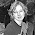## jueves, 18 de octubre de 2012

### IDEA

The IDEA(International Data Encryption Algorithm) was invented by Lai and Massey of Swiss Federal Institute of Technology in 1990. This algorithm is free for distribution in internet and is very popular in United States.

The International Data Encryption Algorithm was invented in Switzerland by the Chinese doctoral Lai and James Massey Xuejia when both worked in the Institute for Signal and Information Processing Federal Institute Swiss Technology in Zurich. It was patented in November 1991.

IDEA has been designed for the purpose of resisting certain attacks on in contrast with DES, which is vulnerable especially to the called "cryptanalysis differentials ". IDEA provides a key size safe enough.

The IDEA is a block cipher that operates on 64-bit sequences each cycle, ie, from 64 bits of plain text, obtained other 64 bits of ciphertext, as determined by a key of 128 bits size, which is more than enough with the current computational power to ensure invulnerability against exhaustive attacks.

The encryption algorithm is based on design concepts which blend operations from different algebraic groups. This property produces the best results against cryptanalysis.

Being a secret key algorithm, the procedure and the key Decryption is the same as that used for encryption. However, the IDEA algorithm is perfectly combinable with other asymmetrical algorithms.

A schematic description of the IDEA is presented next:In each elementary step by passing the text of 64 bits, this suffers from the following alterations to give the encryption:

1. Division of the 64-bit block of text into four sub-blocks of 16 bits: X1, X2, X3 and X4, and 128 of the key 8: Z1 ... Z8. These latter are intentionally more complicated and will be treated separately.
2. Multiplication of X1 for the first subblock of the key Z1
3. To this is added the second subblock X2 and Z2 key
4. To this is added the second subblock X3 and Z3 key
5. That is multiplied by X4 and for four key subblock
6. It is surgically exclusive OR (XOR) between the block result operations of the second and fourth operations
7. XOR between the result of the third and the fifth operation
8. Multiply the results of the sixth to the fifth operation key subblock, Z5
9. It adds the result of the sixth and seventh operations
10. Multiply the result from the ninth to the sixth operation key subblock, Z6
11. Add together the results of the eighth and tenth operation
12. XOR result of the second and the tenth
13. XOR the result of the fourth and tenth
14. XOR result of the third and eleventh
15. XOR the result of the fifth and eleventh
Thus is obtained the ciphertext, joining the four blocks from operations to the last four operations.

Clearly, the security of the cipher text increases when passing several times by the algorithm and perform IDEA 8 times. For feedback, simply swap the second and third blocks of exit and reenter them, in all stages except the last.

After the eighth round, there is a final transformation, which is described in the following four steps:

1. X1 Multiply by the first subblock of the key
2. Add X2 to the second key block
3. Add X3 the third key subblock
4. Multiplying X4 for the fourth subblock key

Finally, concatenate the last four blocks to get the output ciphertext.

The creation of the sub-blocks of the key, in the 1st step of the algorithm, consists in dividing the 128 bits of said key in the eight subblocks which only six are used in the first round, reserving two for second. After this, the key is rotated to the left 25 bits (Using the scroll function of the bits in the word provides the operator "<<"), and again the key is divided into eight subkeys. The first four subkeys are used in the second stage of the algorithm, and the last four in the third. The other key is rotated 25 bits to the left to get the eight following subkeys, and so on.

We use:

• Bitwise exclusive OR (denoted with a circled plus ⊕).
• Addition modulo 216 (denoted with a boxed plus ⊞).
• Multiplication modulo 216+1, where the all-zero word (0x0000) is interpreted as 216 (denoted by a circled dot ⊙).

Example

Message 1001 1100 1010 1100
Key 1101 1100 0110 1111 0011 1111

Encryption

Decryption

Efficiency and safety of IDEA

• IDEA is faster than DES even in software implementations.
• The 128-bit key is more resistant to attacks by "brute force".
• It is argued that IDEA is immune to the "differential cryptanalysis".
• There is evidence of weakness when using only two rounds instead of eight.
• We have discovered some sort of weak keys which could facilitate one chosen plaintext attack. For example, key type
0000 0000 0x00; 0000 0000 000x; xxxx; x000
• IDEA supports operation modes ECB, CBC, CFB and OFB

References:

IDEA
 Imagen

#### 2 comentarios:

1.Esta cosa rechaza mis comentarios...

2.Está bien hecha la tarea, van 7 puntos.